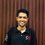# CubeceptionRecently, I was playing with my two year old brother. There was an old $3\times 3$ Rubik's cube lying around and my dad posed a simple problem:

How many selections of $2\times 2$ cubes can be made from a $3\times 3$ Rubik's cube?

The answer to the above question is $8$ and it is so because each corner cube is part of a unique $2\times 2$ selection.

Now, how many selections of $1 \times 1$ cubes can be made from a $3\times 3$? The answer here is too simple and is $27$.

And the number of selections of a $3\times 3$ cube from a $3\times 3$ is $1$.

$1, 8, 27$.... Reminded me of something. This note is an attempt to prove or disprove the following hypothesis.

In an $n \times n$ Rubik's cube, the number of selections of solid cubes of edge length $m(\le n)$ is $(n-m+1)^3$

I am convinced that there is an elegant solution to this problem and that the hypothesis is true.Note by Raghav Vaidyanathan
5 years, 10 months ago

This discussion board is a place to discuss our Daily Challenges and the math and science related to those challenges. Explanations are more than just a solution — they should explain the steps and thinking strategies that you used to obtain the solution. Comments should further the discussion of math and science.

When posting on Brilliant:

• Use the emojis to react to an explanation, whether you're congratulating a job well done , or just really confused .
• Ask specific questions about the challenge or the steps in somebody's explanation. Well-posed questions can add a lot to the discussion, but posting "I don't understand!" doesn't help anyone.
• Try to contribute something new to the discussion, whether it is an extension, generalization or other idea related to the challenge.

MarkdownAppears as
*italics* or _italics_ italics
**bold** or __bold__ bold
- bulleted- list
• bulleted
• list
1. numbered2. list
1. numbered
2. list
Note: you must add a full line of space before and after lists for them to show up correctly
paragraph 1paragraph 2

paragraph 1

paragraph 2

[example link](https://brilliant.org)example link
> This is a quote
This is a quote
    # I indented these lines
# 4 spaces, and now they show
# up as a code block.

print "hello world"
# I indented these lines
# 4 spaces, and now they show
# up as a code block.

print "hello world"
MathAppears as
Remember to wrap math in $$ ... $$ or $ ... $ to ensure proper formatting.
2 \times 3 $2 \times 3$
2^{34} $2^{34}$
a_{i-1} $a_{i-1}$
\frac{2}{3} $\frac{2}{3}$
\sqrt{2} $\sqrt{2}$
\sum_{i=1}^3 $\sum_{i=1}^3$
\sin \theta $\sin \theta$
\boxed{123} $\boxed{123}$

Sort by:

Consider one dimension first. Given an array of length $n$, we can place a smaller array of length $m$ in $n-m+1$ positions. This is the same for all three dimensions, bringing the total number of placings to be $(n+m-1)^3$.

- 5 years, 10 months ago

Great explanation! Thanks!

- 5 years, 10 months ago